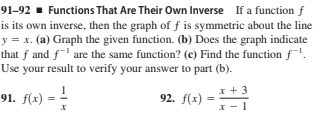# 91–92 ▪ Functions That Are Their Own Inverse If a function f is its own inverse, then the graph of f is symmetric about the line y = x. (a) Graph the given function. (b) Does the graph indicate that f and f' are the same function? (c) Find the function f. Use your result to verify your answer to part (b). 91. f(x) 92. f(x) x +3

Questionhelp_outlineImage Transcriptionclose91–92 ▪ Functions That Are Their Own Inverse If a function f is its own inverse, then the graph of f is symmetric about the line y = x. (a) Graph the given function. (b) Does the graph indicate that f and f' are the same function? (c) Find the function f. Use your result to verify your answer to part (b). 91. f(x) 92. f(x) x +3 fullscreen

### Want to see this answer and more?

Experts are waiting 24/7 to provide step-by-step solutions in as fast as 30 minutes!*

*Response times may vary by subject and question complexity. Median response time is 34 minutes for paid subscribers and may be longer for promotional offers.
Tagged in
Math
Calculus

### Other